Perl ProgrammingLearn about Operators in Perl Programming

# Learn about Operators in Perl Programming

After talking about the first type of variables: Scalars, it is time to know what to do with those variables. Ready to join us?! Great!! Here we go.

* * * * * *

What are Operators?
Operations need Operators. So to perform an arithmetic operation, we need an arithmetic operator. Similarly, to perform a logical operation, a logical operator is needed.
Perl supports several types of operators. The following sections will present with details those types, and illustrate them with examples.

Arithmetic Operators
A computer is named so because it computes (makes computations). So, you will never encounter a computer programming language that doesn’t support arithmetic operators. This is as far as I know (I have worked on Pascal, C, C++, Visual Basic, Visual C++, Java, Python, Shell Scripting, and C#).

The following table lists the arithmetic operators supported in Perl:

 Operator Description + Adds two numbers – Subtracts the second operand from the first * Multiplies two numbers / Divides the left side operand by the right side operand % Divides the left side operand by the right side operand and returns the remainder ** Raises the left side operand to the power of the right side operand

Example
The following script prompts the user to enter two numbers, adds them, then prints the results on the standard output.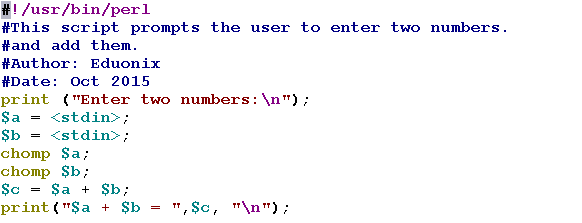Let’s see how this script will behave: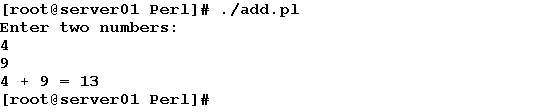Now, to the explanation: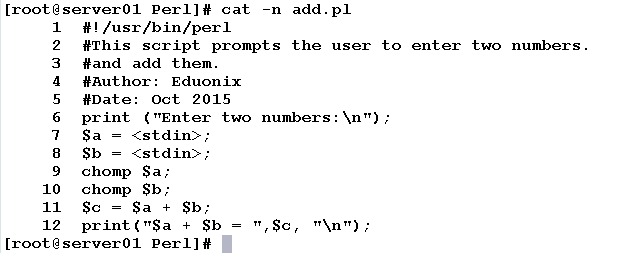• Line 1: The Shebang. It tells the program loader to use the /usr/bin/perl to interpret this script file.
• Line 6: prints a message to the user asking him/her to enter two numbers.
• Lines 7 and 8: read the user inputs and stores them in variables \$a and \$b.
• Line 9 and 10: remove the trailing newline character from both \$a and \$b.
• Line 11: performs the addition operation using the ‘+’ operator, and stores the result in \$c.
• Line 12: prints the result to the user.

So simple and straightforward.
Let’s take another bit more complicated one.

Example
We need to write a script that calculates the volume of a sphere given its radius.

Hint: for a sphere with radius R, its volume could be calculated using the formula:

Volume = 4/3 π R3

Consider the following script.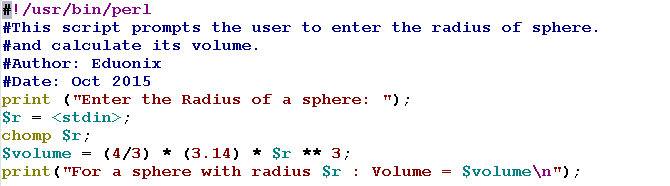Now, execute the script: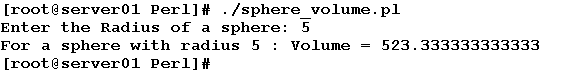Although the script is self-explanatory, I would like to draw your attention to the line that contains the mathematical operation:

`\$volume = (4/3) * 3.14 * \$r ** 3;`

This line divides 4 by 3, then multiplies the result by 3.14 (the mathematical constant for the approximate ratio). Again, the result is multiplied by the result of raising the radius to the power of 3. The final result is the required volume.Assignment Operators
– Assignment Operators?! Isn’t it just the normal one “=”?!

No, besides to the ordinary assignment operator “=”, there are several ones supported in the Perl language. They are all listed in the following table:

 Operator Description = Ordinary Assignment operator. += Adds the right side operand to the left side one, and assigns the result to the left side operand. -= Subtracts the right side operand from the left side one, and assigns the result to the left side operand. *= Multiplies the right side operand by the left side one, and assigns the result to the left side operand. /= Divides the left side operand by the right side one, then assigns the result to the left side operand. **= Raises the left side operand to the power of the right side one, and then assigns the result to the left side operand.

Example
Consider:

`\$counter = 0;`

The following statement will increment the \$counter variable by 1:

`\$counter += 1;`

This is exactly equivalent to saying:

`\$counter = \$counter + 1;`

Example
The following script accepts three numbers from the user and calculates their total.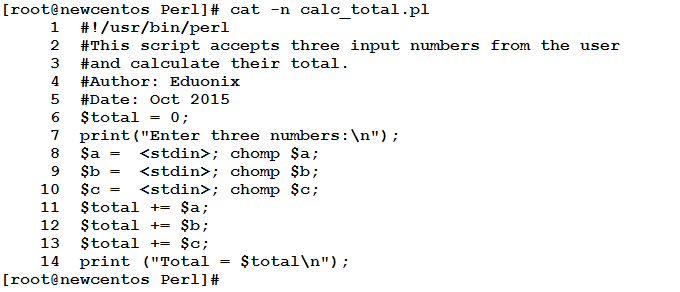Let’s execute it and see what we get: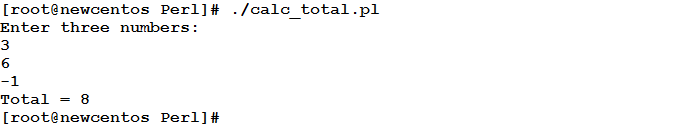Although this problem could be solved using one statement that adds the three variables together and assigns their total to the variable \$total, I wrote it this way to illustrate the meaning of arithmetic assignment operator.

Another point that deserves mentioning is the use of more than one statement in one line. In general, you could write several statements together in one line this way:

`statement1; statement2; statement3; statement4;`

Increment / Decrement Operators
If you have some knowledge of C/C++ or Java, you may know the ++ and -– operators. The same two operators with their C/C++ meaning are supported also in Perl.

Increment Operator ++
When used directly after a variable, the ++ operator increments the value of the variable by 1.

Decrement Operator
The — operator decrements the value of variable by 1.

Example
Again, we need to increment a counter \$counter by 1. We could use the normal way:

`\$counter = \$counter + 1;`

Or:

`\$counter += 1;`

Or, use the auto increment operator:

`\$counter++;`

The Range Operator “..”
Given two numeric operands x and y, the Range operator returns the numbers in the range from x to y by a step of 1, inclusively. You will see how this operator is useful when we talk about loops. (That looks similar to the seq command in Linux)

Syntax

`(x..y)`

Example
To print the sequence of numbers from 1 to 6

`(1..6)`

* * * * * *

Summary

• Operators are necessary for performing various operations.
• Perl supports several types of operators: arithmetic, comparison, assignment, logical, etc.
• Arithmetic operators enables the developer to perform basic numeric calculations like addition, subtraction, multiplication, division, and expontiation.
• Besides to the normal assignment operator ‘=’ , Perl supports a list of arithmetic assignment operators.
• Like C/C++, Perl supports the increment and decrement operators (++ and –)

That was part one in the topic of Operators. The next is part two that will talk about comparison, logical, and string operators.
See you there.

### Top 10 cyber security threats that arose last year

21,501Fans
4,218Followers
103,000Subscribers

### Top 10 cyber security threats that arose last year

- Advertisement -